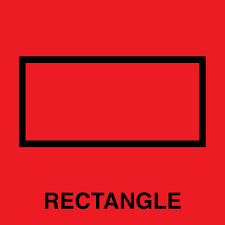# The perimeter of a rectangle?

General Information - member notified that some attempt must be shown
Homework Statement:
A rectangle has a ratio of 3:2. The area is 294 dm2. What is the perimeter?
Relevant Equations:
:

A rectangle has a ratio of 3:2. The area is 294 dm2. What is the perimeter?

How am I supposed to calculate this?

ehild
Homework Helper
How am I supposed to calculate this?
What is a rectangle? How do you calculate the area and the perimeter of a rectangle?So the area and ratio are given ( 3:2) and 3 represents the long side and 2 the shorter side. I calculate the area like this: A = L * W ( L is the length and W is the width). I think that the perimeter is 2*W+2*L

Mark44
Mentor
View attachment 243519 So the area and ratio are given ( 3:2) and 3 represents the long side and 2 the shorter side. I calculate the area like this: A = L * W ( L is the length and W is the width). I think that the perimeter is 2*W+2*L
But you know that the ratio of the length to the width is 3/2, or ##\frac L W = \frac 3 2##. From this equation you can write the length in terms of the width.

Write your area equation using the value given, and write the equation for the perimeter. Keep in mind what I wrote above.

Chestermiller
Mentor
Let L=3x and W=2x, where x is unknown.